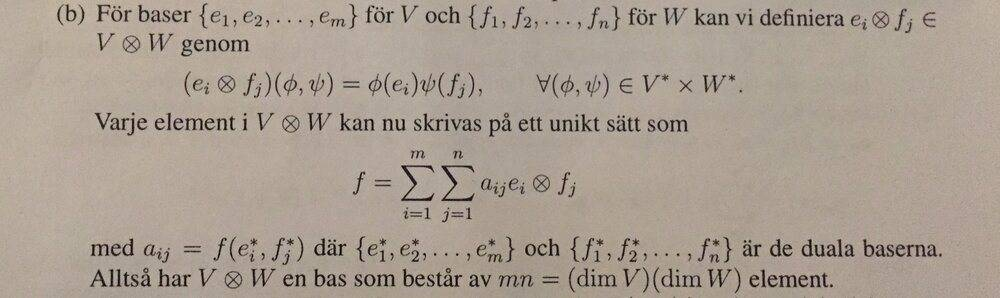# Prove that dim(V⊗W)=(dim V)(dim W)

• I
• Karl Karlsson

#### Karl Karlsson

TL;DR Summary
This proof was in my book.
(see image below)
Tensor product definition according to my book: $$V⊗W=\{f: V^*\times W^*\rightarrow k | \textrm {f is bilinear}\}$$ wher ##V^*## and ##W^*## are the dual spaces for V and W respectively.

I don't understand the step where they say ##(e_i⊗f_j)(φ,ψ) = φ(e_i)ψ(f_j)##. Why is this equality true? What definition has been used? My understanding for all of this is still quite basic.
This proof was in my book.Tensor product definition according to my book: $$V⊗W=\{f: V^*\times W^*\rightarrow k | \textrm {f is bilinear}\}$$ wher ##V^*## and ##W^*## are the dual spaces for V and W respectively.

I don't understand the step where they say ##(e_i⊗f_j)(φ,ψ) = φ(e_i)ψ(f_j)##. Why is this equality true? What definition has been used? My understanding for all of this is still quite basic.

This is true because they define it that way. By definition, ##e_i \otimes f_j## is the bilinear map defined by $$e_i \otimes f_j: V^* \times W^* \to k: (\phi, \psi) \mapsto \psi(e_i) \psi(f_j)$$
$$T\in V\otimes W \Longleftrightarrow T=\sum_{\rho=1}^R v_{\rho} \otimes w_{\rho} \, : \,(X,Y)\longmapsto \sum_{\rho=1}^R v_{\rho}(X) \cdot w_{\rho}(Y) \in k$$
••